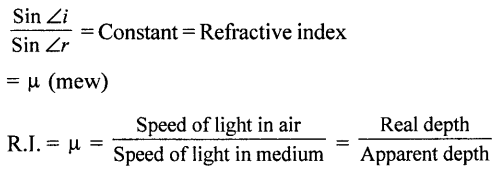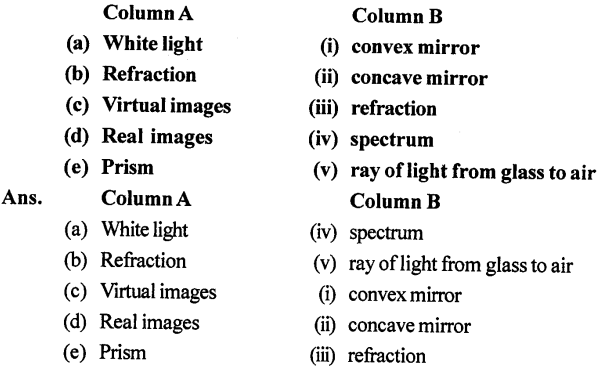## Selina Concise Physics Class 8 ICSE Solutions – Light Energy

ICSESolutions.com provides step by step solutions for Selina Concise ICSE Solutions for Class 8 Physics Chapter 5 Light Energy. You can download the Selina Concise Physics ICSE Solutions for Class 8 with Free PDF download option. Selina Publishers Concise Physics for Class 8 ICSE Solutions all questions are solved and explained by expert teachers as per ICSE board guidelines.

Selina Concise ICSE Solutions for Class 8 Physics Chapter 5 Light Energy

• Light is a form of energy which produces in us the sensation of sight i.e. we can see objects only when light falls on them and then reflected into our eye.
•  Velocity of light in air or in vacuum is 300000 km per second.
Or
3 x 10ms-1
• As light passes into different mediums its speed changes and depends upon the density of medium i.e. it decreases with increase in density i.e. it is 2.25 × 108 m/s in water and 2 x 108 ms-1 in glass as water is
denser than air ( $$_{ w }^{ a }{ \mu }$$ = 1.33 ) and glass is still optically denser than water
( $$_{ g }^{ a }{ \mu }$$ =1.5 ) i.e. slower in water and still slower in glass.
• Light travels in a straight line.
• As light travels from one transparent medium to other transparent medium and falls oblique at another medium, its path changes and this change in path is called REFRACTION OF LIGHT.
•  When ray of light travels from RARER (less-denser) to DENSER medium, it bends TOWARD the normal AND when it travels from a DENSER to a RARER medium it bends away from NORMAL
•  ANGLE of INCIDENCE : “The angle which incident ray makes with normal”. “∠i”
•  ANGLE OF REFRACTION: “The angle which refracted ray makes with normal” “ ∠r ”
∠i is not equal to ∠r
•  LAWS OF REFRACTION or SNELL’S LAWS OF REFRACTION:
(i) Incident ray, normal at the point of incidence and Refracted ray all lie in the same plane.
(ii) Ratio of sine of angle of incidence to the sine of angle of refraction is constant.
••  EFFECTS OF REFRACTION :
(i) A coin placed in water appears to be raised.
(ii) Swimming pool seen from above appears SHADOW.
(iii) A pencil in water appears to be bent.
(iv) MIRAGE in desert, EARLY Sunrise, LATE SUN set are all due to REFRACTION of light.
• White light is a band of seven colours-VIBGYOR. Speed of all colours of the white light in AIR or VACUUM is same, but different different transparent mediums.
•  In glass or water Speed of VIOLET colour is MINIMUM and speed of RED light is MAXIMUM
• Refractive index of medium is minimum for VIOLET light and R.I. of medium is maximum for red light.
• DISPERSION: “The splitting (breaking) of white light into seven colours is called DISPERSION OF LIGHT.
•  CAUSE OF DISPERSION: Speed of different colours is different in glass or water and different colours get separated from each other on refraction at second surface of glass prism.

Test yourself

A. Objective Questions

1. Write true or false for each statement

(a) Water is optically denser than glass.
Water is optically denser than air.

(b) A ray of light when passes from glass to air, bends towards the normal.

(c) The speed of light is more in glass than in water.

(d) The depth of a pond when seen from above appears to be less.

(e) Light travels at a lower speed in water than in air.

(f) Light travels in the same straight line path while passing through different media.

(g) The angle formed between the normal and the refracted ray is known as the angle of incidence.

(h) At the point of incidence, a line drawn at right angles to the surface, separating the two media, is called the normal.

(i) Image is formed by a mirror due to refraction of light.

(j) Rays of light incident parallel to the principal axis pass through the focus after reflection from a concave mirror.

(k) A convex mirror is used as a shaving mirror.

(l) The focal length of a convex mirror is equal to its radius of curvature.

(m) A concave mirror converges the light-rays, but a convex mirror diverges them.

(n) A virtual image formed by a spherical mirror is always erect and situated behind the mirror.

2. Fill in the blanks

(a) Water is optically denser than air.
(b) Air is optically rarer than glass.
(c) When a ray of light travels from water to air, it bends away from the normal.
(d) When a ray of light travels from air to glass, it bends towards the normal.
(e) When white light passes through a prism, it disperses
(f) The splitting of white light into its constituent colours is called dispersion.
(g) A concave mirror is obtained on silvering the outer surface of a part of a hollow glass sphere.
(h) Radius of curvature of a spherical mirror is two times its focal length.
(i) The angle of incidence for a ray of light passing through the centre of curvature of a spherical mirror is
(j) A convex mirror always forms a virtual image.
(k) A concave mirror forms a virtual image for an object placed between pole and focus.

3. Match the following4. Select the correct alternative

(a) The speed of light in air or vacuum is

1. 3 × 10M s-1
2.  2.25 × 108 m s-1
3.  332 ms-1
4.  2.0 × 108 ms-1

(b) A ray of light moving from an optically rarer to a denser medium

1.  bends away from the normal
2.  bends towards the normal
3.  remains undeviated
4.  none of the above

(c) The angle between the normal and refracted ray is called

1.  angle of deviation
2.  angle of incidence
3.  angle of refraction
4.  angle of emergence.

(d) The property of splitting of white light into its seven constituent colours is known as

1.  rectilinear propagation
2.  refraction
3.  reflection
4.  dispersion

(e) The seven colours in the spectrum of sunlight in order, are represented as :

1.  VIBGYOR
2.  VIGYBOR
3.  BIVGYOR
4.  RYOBIVG

(f) A ray of light passing through centre of curvature of a spherical mirror, after reflection

1. passes through the focus
2.  passes through the pole
3.  becomes parallel to the principal axis
4.  retraces its own path.

(g) If the radius of curvature of a concave mirror is 20 cm, its focal length is:

1.  10 cm
2.  20 cm
3.  40 cm
4.  80 cm

(h) The image formed by a convex mirror is

1.  erect and diminished
2.  erect and enlarged
3.  inverted and diminished
4.  inverted and enlarged.

(i) The image formed by a concave mirror is of the same size as the object, if the object is placed

1. at the focus
2. between the pole and focus
3.  between the focus and centre of curvature
4.  at the centre of curvature.

(j) A convex mirror is used

1.  as a shaving mirror
2.  as a head mirror by a dentist
3.  as a rear view mirror by a driver
4.  as a reflector in torch.